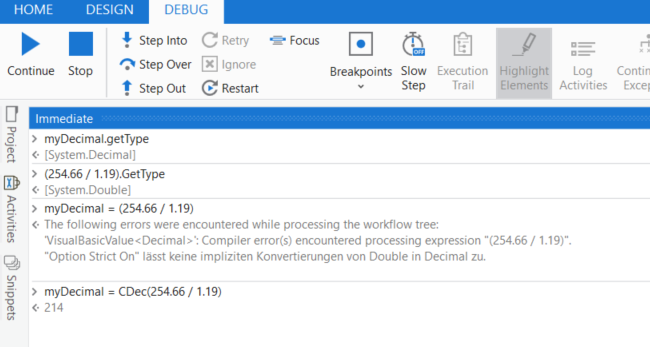# Divide decimal by decimal

Trying to divide a decimal by a decimal for currency exchange.

E.g Total decimal value 254.66 / 1.19 (to find euro conversion) I get the error - Option Strict On disallows implicit conversions from ‘Double’ to ‘Decimal’.

How would I write this code to divide a decimal by another decimal?

Thanks

Hey!

Try this

``````cdbl(Variable)
``````

Regards,
NaNi

That doesn’t work unfortunatelyHi,

FYI, if you want to handle some value as Decimal, please add `d` just after the numeric, as the following.

``````1.19d

254.66d / 1.19d
``````

Regards,

1 Like

This worked, thank you

This topic was automatically closed 3 days after the last reply. New replies are no longer allowed.